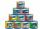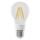Sequence

In the arithmetic sequence is a1=-1, d=4. Which member is equal to the number 203?

Result

n =  52

Solution:Leave us a comment of example and its solution (i.e. if it is still somewhat unclear...):Be the first to comment!To solve this example are needed these knowledge from mathematics:

Do you have a linear equation or system of equations and looking for its solution? Or do you have quadratic equation?

Next similar examples:

1. SequenceWrite the first 7 members of an arithmetic sequence: a1=-3, d=6.
2. Nineteenth memberFind the nineteenth member of the arithmetic sequence: a1=33 d=5 find a19
3. SequenceBetween numbers 1 and 53 insert n members of the arithmetic sequence that its sum is 702.
4. Sequence 2Write the first 5 members of an arithmetic sequence a11=-14, d=-1
5. Sequence 3Write the first 5 members of an arithmetic sequence: a4=-35, a11=-105.
6. SequenceWrite the first 6 members of these sequence: a1 = 5 a2 = 7 an+2 = an+1 +2 an
7. AS sequenceIn an arithmetic sequence is given the difference d = -3 and a71 = 455. a) Determine the value of a62 b) Determine the sum of 71 members.
8. TreesA certain species of tree grows an average of 0.5 cm per week. Write an equation for the sequence that represents the weekly height of this tree in centimeters if the measurements begin when the tree is 200 centimeters tall.
9. 6 termsFind the first six terms of the sequence. a1 = 7, an = an-1 + 6
10. AP - simpleDetermine the first nine elements of sequence if a10 = -1 and d = 4
11. CansHow many cans must be put in the bottom row if we want 182 cans arrange in 13 rows above so that each subsequent row has always been one tin less? How many cans will be in the top row?
12. SeatsSeats in the sport hall are organized so that each subsequent row has five more seats. First has 10 seats. How many seats are: a) in the eighth row b) in the eighteenth row
13. Fifth of the numberThe fifth of the number is by 24 less than that number. What is the number?
14. Walnutsx walnuts were in the mission. Dano took 1/4 of nuts Michael took 1/8 from the rest and John took 34 nuts. It stayed here 29 nuts. Determine the original number of nuts.
15. Theorem proveWe want to prove the sentence: If the natural number n is divisible by six, then n is divisible by three. From what assumption we started?
16. One percentOne percent of all the lights in the city are LED, the remaining 99% are conventional. Other types are not there. John counted them honestly but he had counted only conventional. After a good dinner he registered numbers and he have notice that from all t
17. Mom and daughterMother is 39 years old. Her daughter is 15 years. For many years will mother be four times older than the daughter?